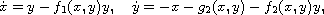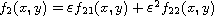Electron. J. Differential Equations, Vol. 2016 (2016), No. 318, pp. 1-22.

### Upper bounds for the number of limit cycles of polynomial differential system Selma Ellaggoune, Sabrina Badi

Abstract:
Forsmall we consider the number of limit cycles of the polynomial differential systemwhere,andwherehave degreerespectively for each. We provide an accurate upper bound of the maximum number of limit cycles that this class of systems can have bifurcating from the periodic orbits of the linear centerusing the averaging theory of first and second order. We give an example for which this bound is reached.

Submitted October 1, 2016. Published December 14, 2016.
Math Subject Classifications: 34C25, 34C29, 37G15.
Key Words: Limit cycle; Lienard differential equation; averaging method.

Show me the PDF file (238 KB), TEX file for this article.Selma Ellaggoune Laboratory of Applied Mathematics and Modeling University 8 Mai 1945 Guelma, Algeria email: sellaggoune@gmail.com Sabrina Badi Laboratory of Applied Mathematics and Modeling University 8 Mai 1945 Guelma, Algeria email: badisabrina@yahoo.fr